# Generate Recursive Mazes

Supports the generation of parallelogram, equilateral triangle, regular hexagon, isosceles trapezoid, Koch snowflake, 'hexaflake', Sierpinski triangle, Sierpinski carpet and Sierpinski trapezoid mazes via 'TurtleGraphics'. Mazes are generated by the recursive method: the domain is divided into sub-domains in which mazes are generated, then dividing lines with holes are drawn between them, see J. Buck, Recursive Division, < http://weblog.jamisbuck.org/2011/1/12/maze-generation-recursive-division-algorithm>.

Generate mazes recursively via Turtle graphics.

-- Steven E. Pav, [email protected]

## Installation

This package can be installed from CRAN, via drat, or from github:

# parallelogram maze

The simplest maze to generate recursively is a parallelogram. One can generate a parallelogram maze by splitting the domain into two parts by an arbitrary cut line with a hole in it, and then recursively creating mazes on both parts. Unlike some shapes, this method applies for arbitrary (integral) side lengths, where by 'length' we mean in units of 'hallway widths', what we call the `unit_len` in the API. Here is a simple parallelogram maze: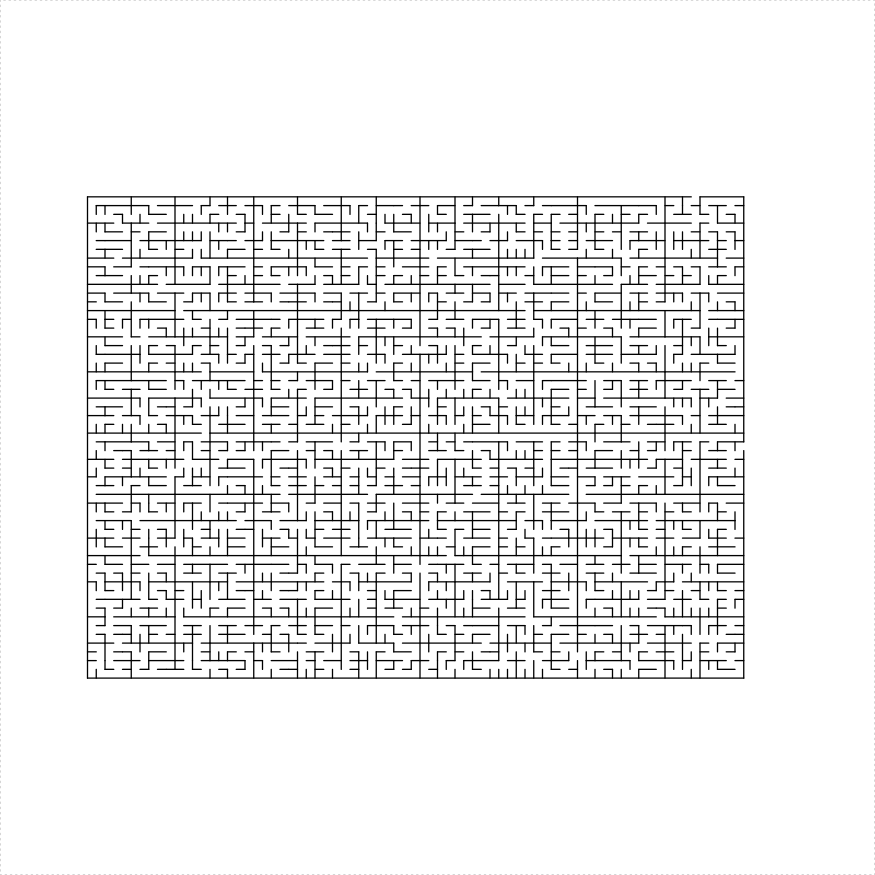The `parallelogram_maze` function admits a `balance` parameter which controls how the maze should be recursively subdivided. A negative value creates imbalanced mazes, while positive values create more uniform mazes. In the example below we create seven mazes side by side with an increasing balance parameter: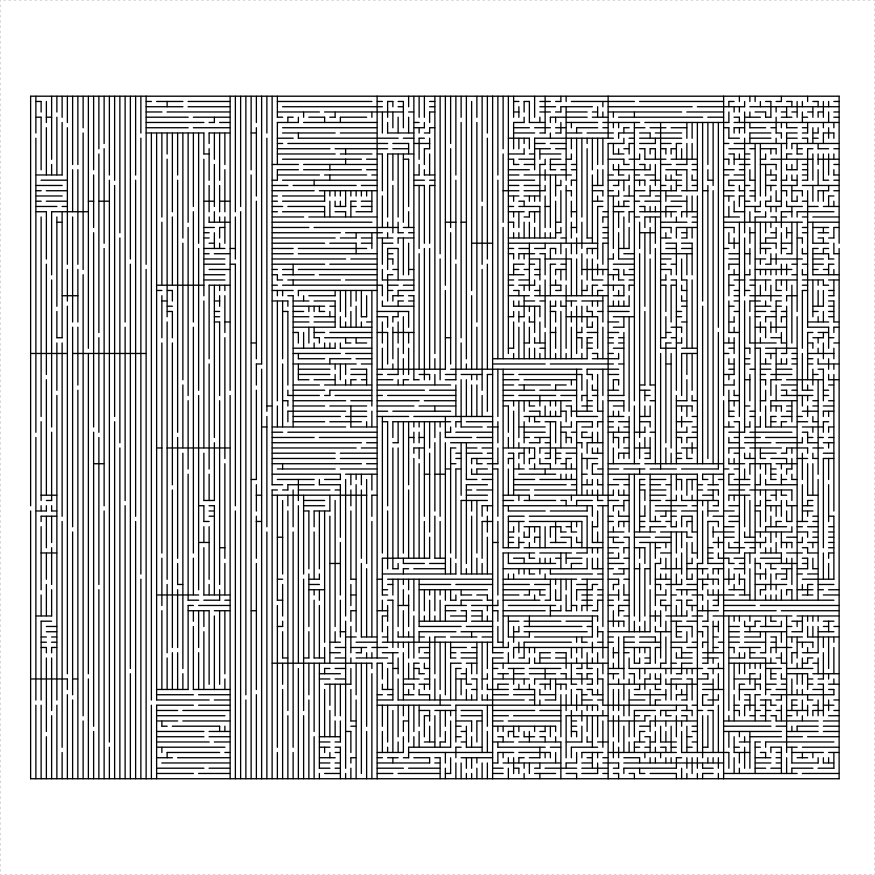# triangle maze

An equilateral triangle maze can be constructed in a number of different ways:

1. Create four equilateral mazes with lines with holes between them. This only works if the side length of the original is a power of two.
2. Cut out a parallelogram and attach two equilateral triangles. Again only if the side length is a power of two.
3. Create an isosceles trapezoid maze, then stack an equilateral triangle on top of it. This only works if the side length is even.
4. Create a regular hexagonal maze and three equilateral mazes in the corners. This only works if the side length of the original triangle is divisible by three.
5. Shave off a single hallway and create an equilateral triangular maze of side length one less than the original.

I illustrate them here: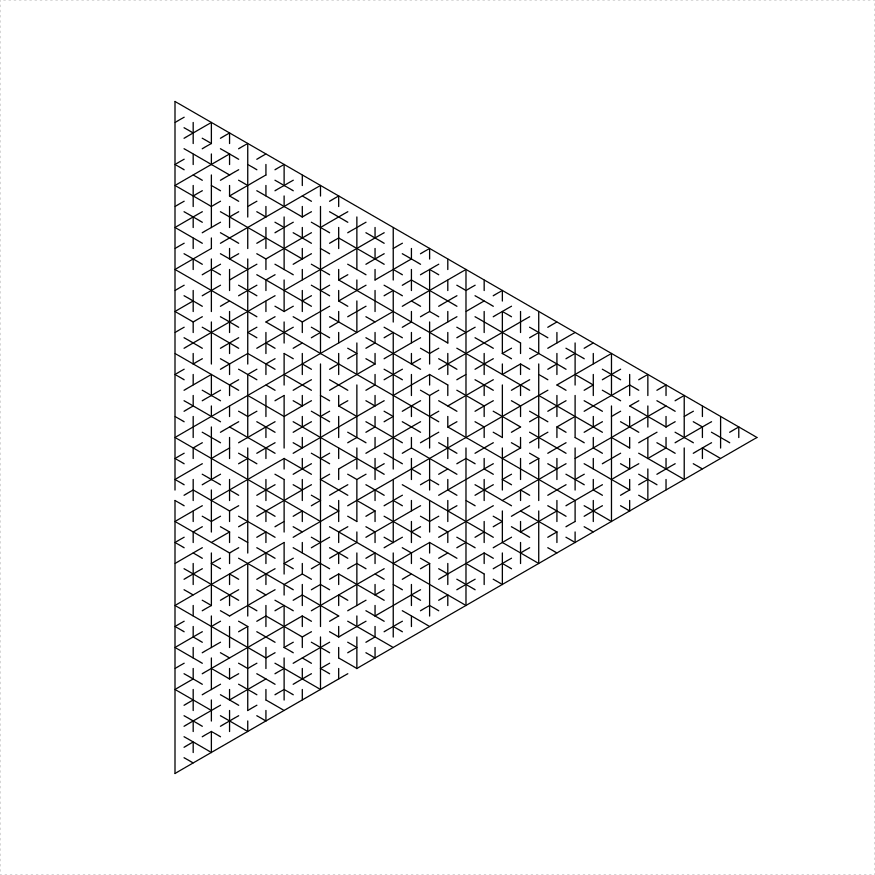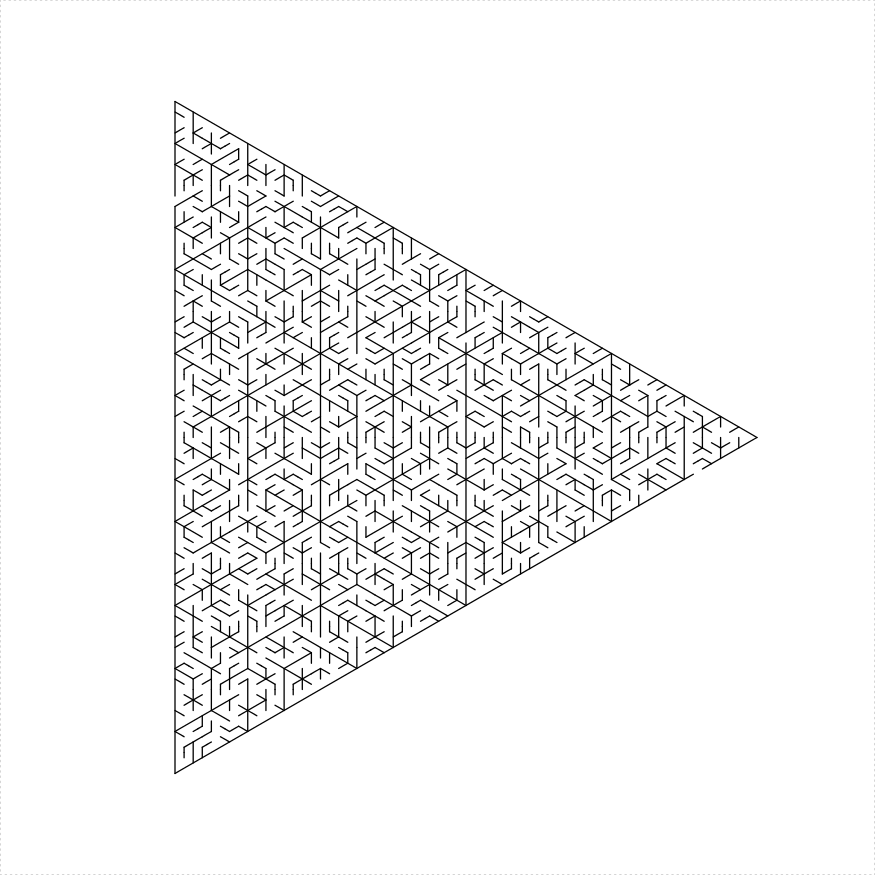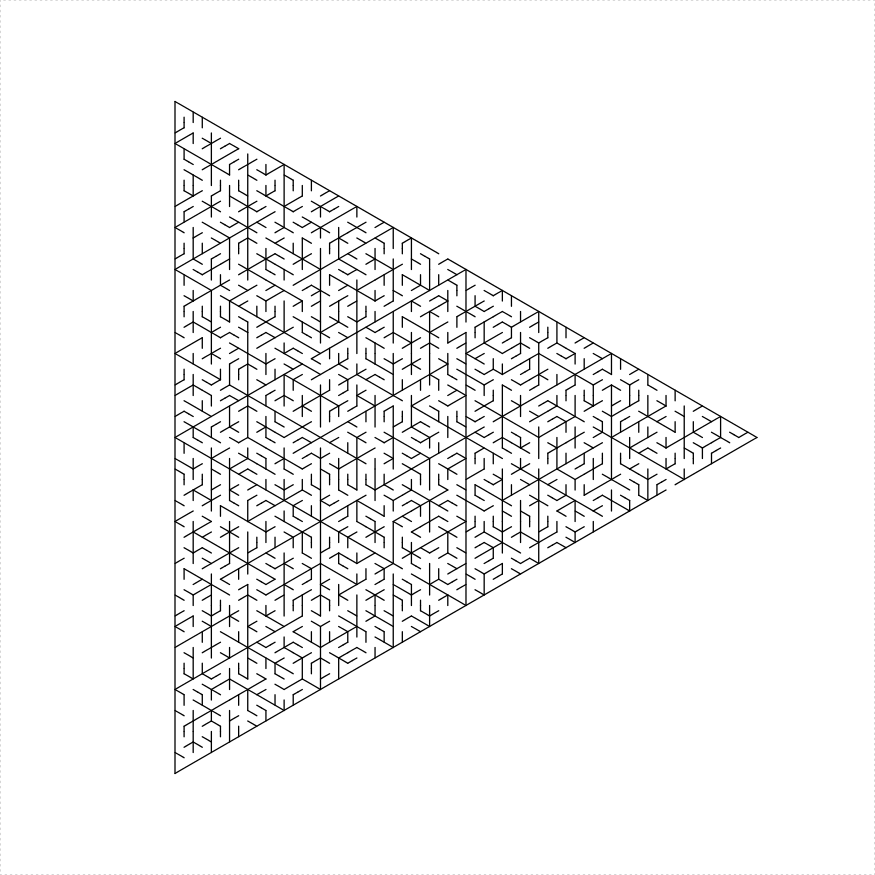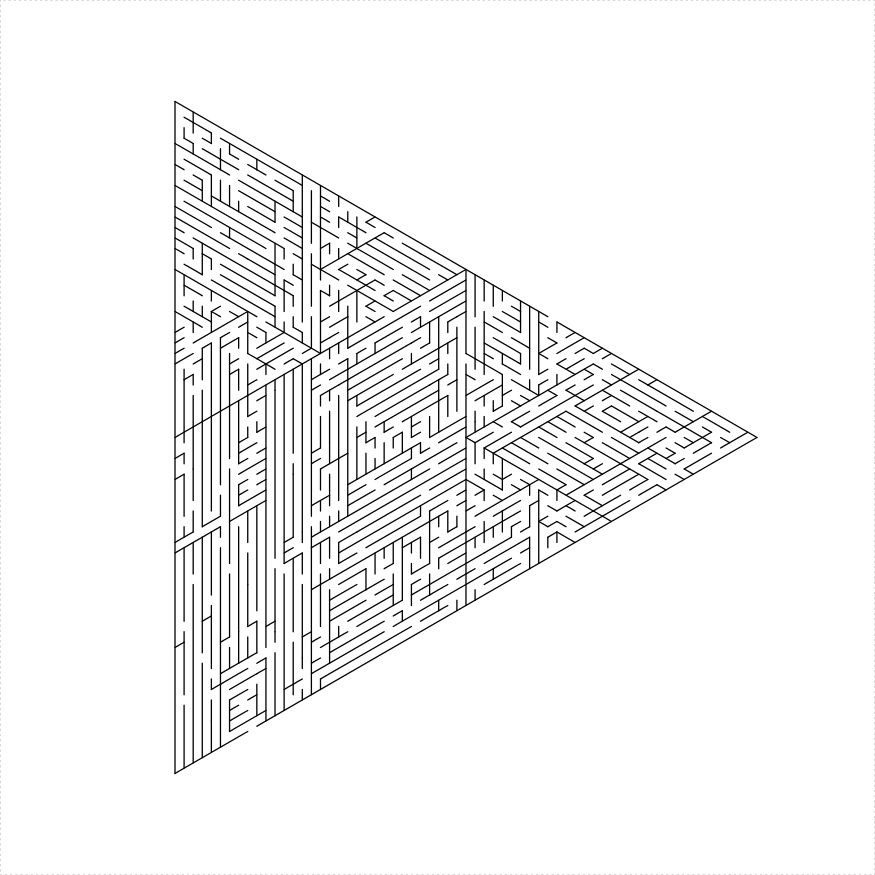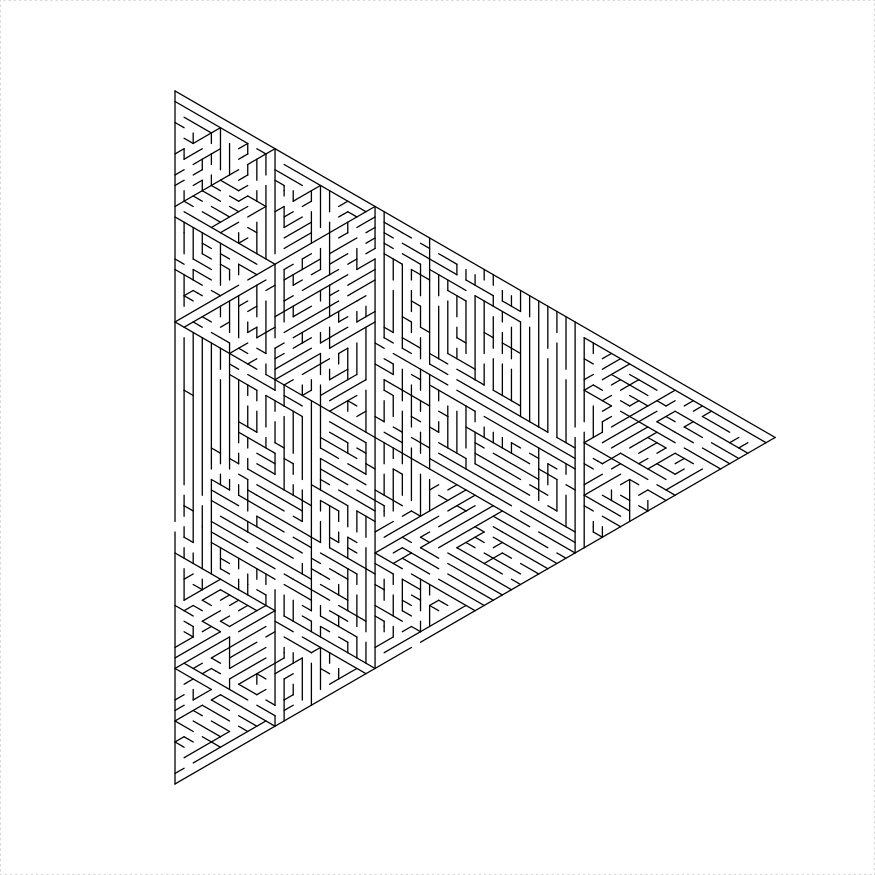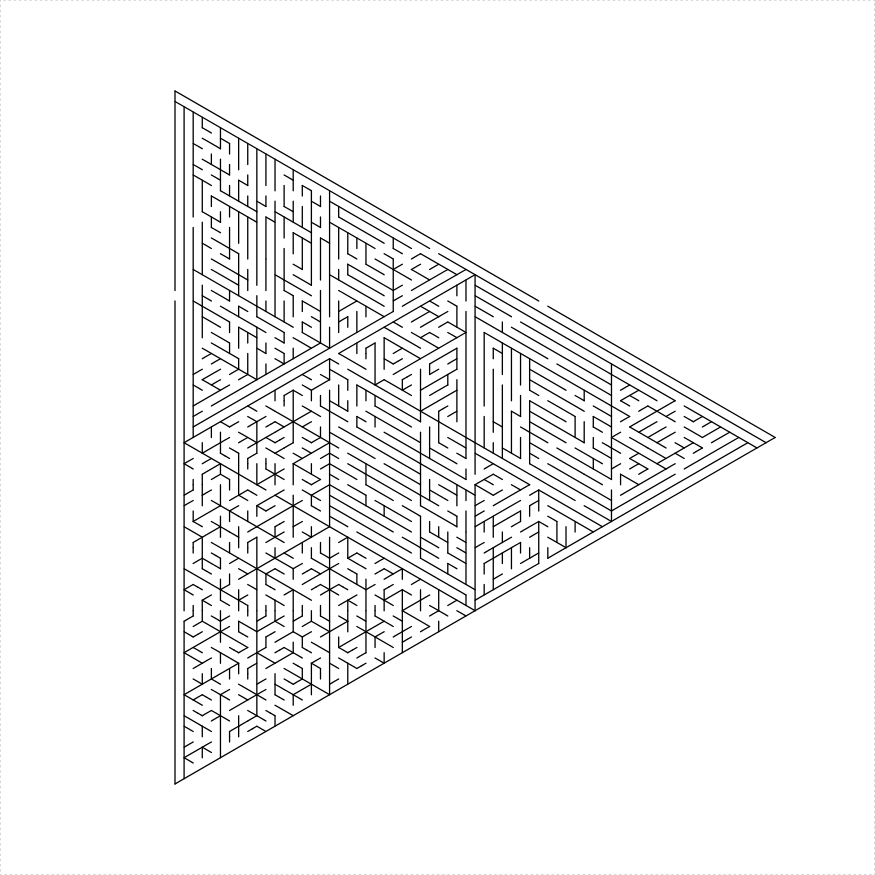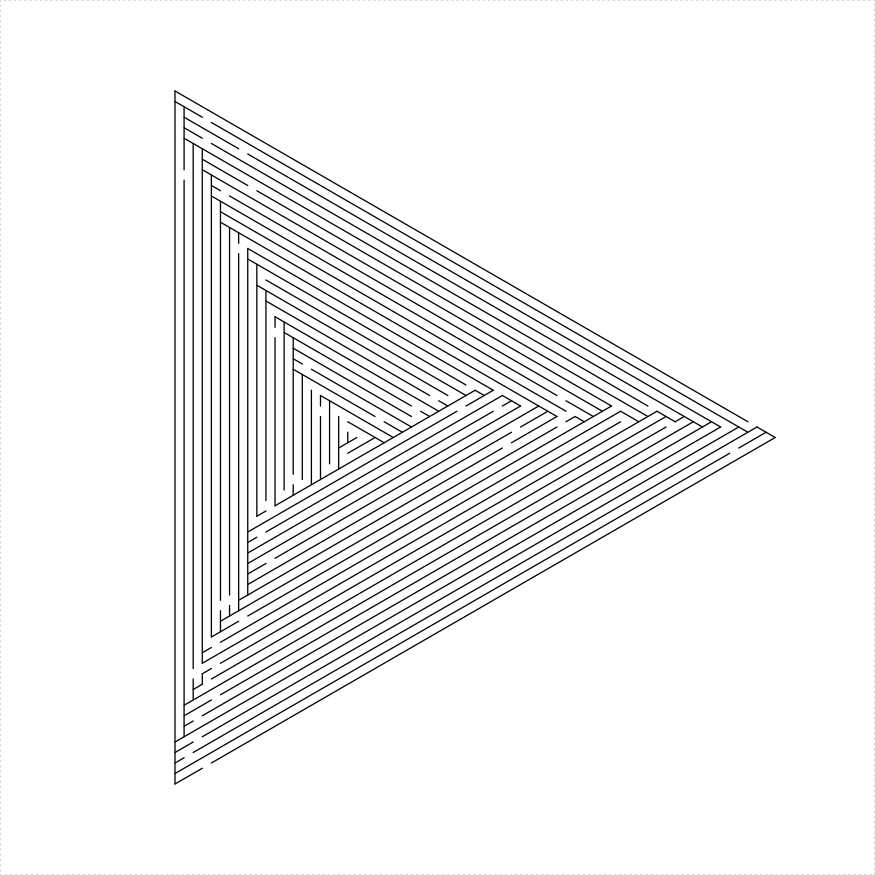# hexagon maze

An regular hexagonal maze can be constructed in a number of different ways:

1. Decompose the hexagon as 6 equilateral triangle mazes, with one solid line and five lines with holes dividing them.
2. Create two isosceles trapezoid mazes with long sides joined by a line with a hole.
3. Create three parallelogram mazes with one solid line and two lines with holes dividing them.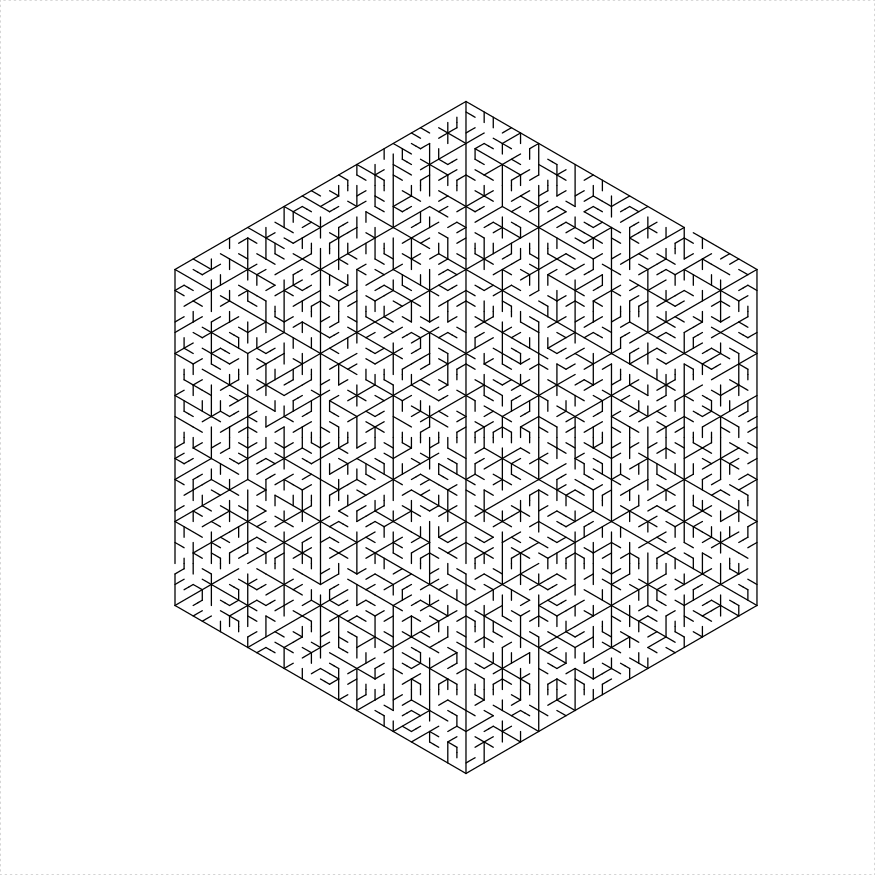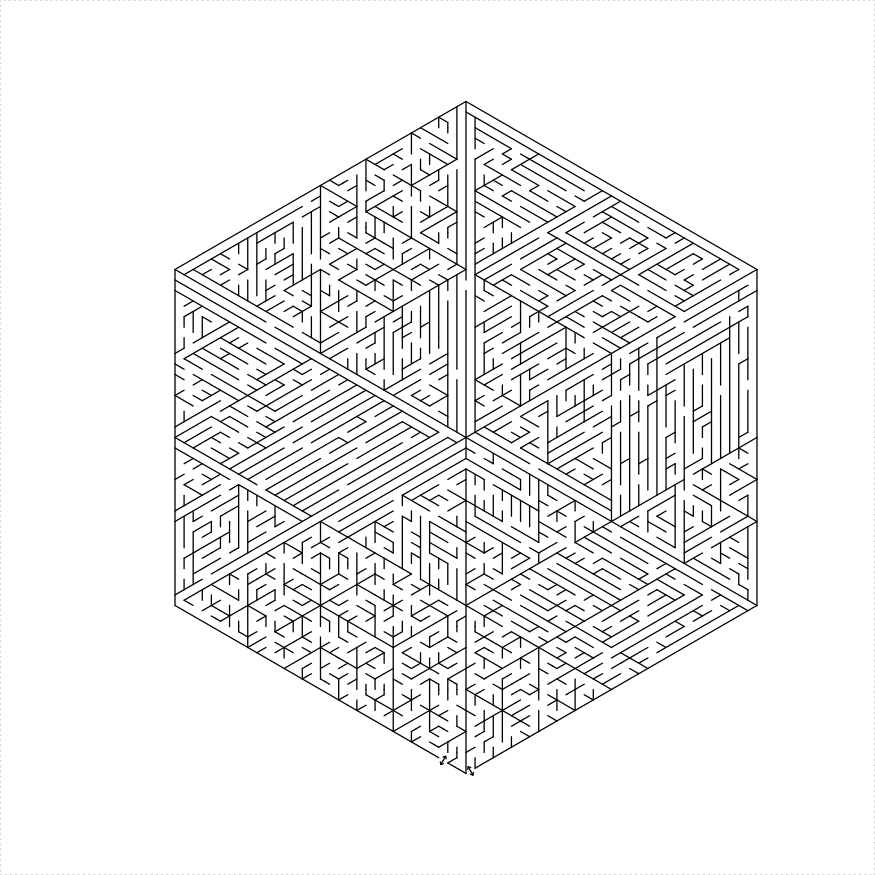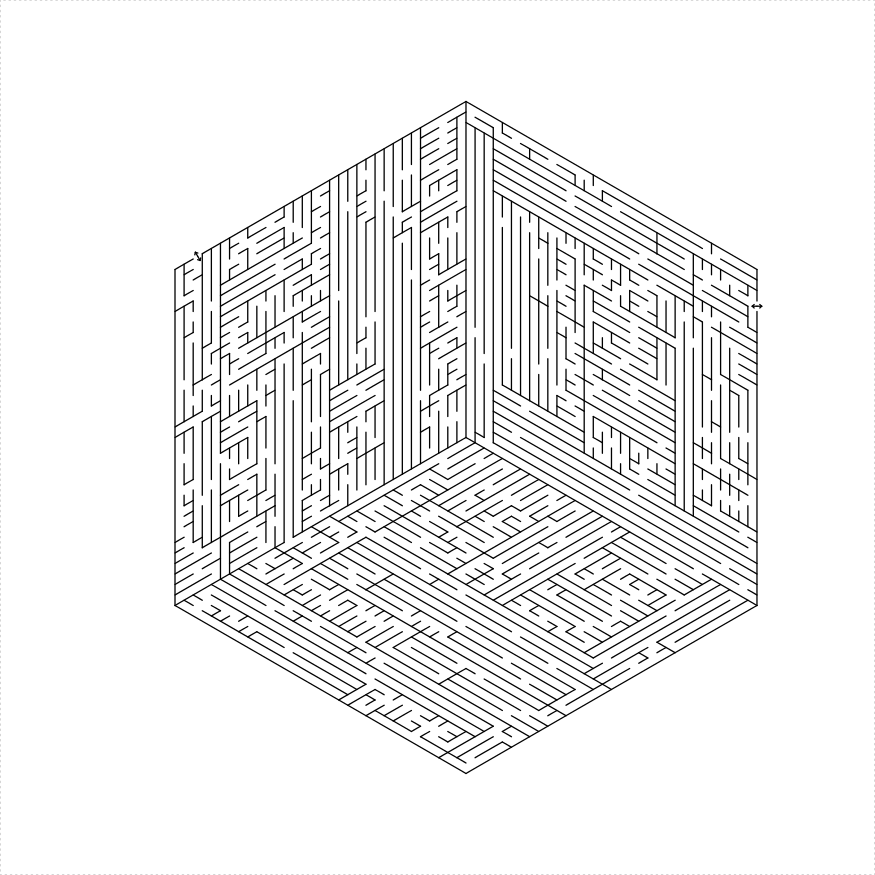# dodecagon maze

A dodecagon can be dissected into a hexagon and a ring of alternating squares and equilateral triangles: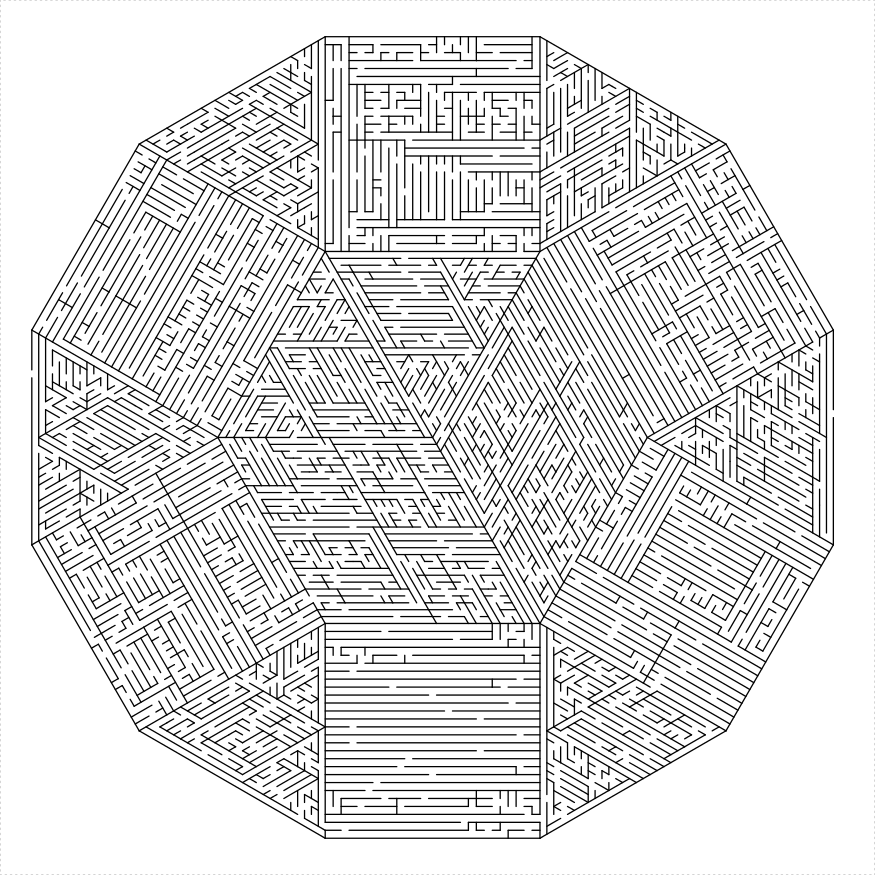# trapezoid maze

An isosceles trapezoid maze can be constructed in a number of different ways:

1. Decompose as four trapezoidal mazes with a 'bone' shape between them consisting of two solid lines and three lines with holes.
2. Decompose as a parallelogram and an equilateral triangle with a line with holes between them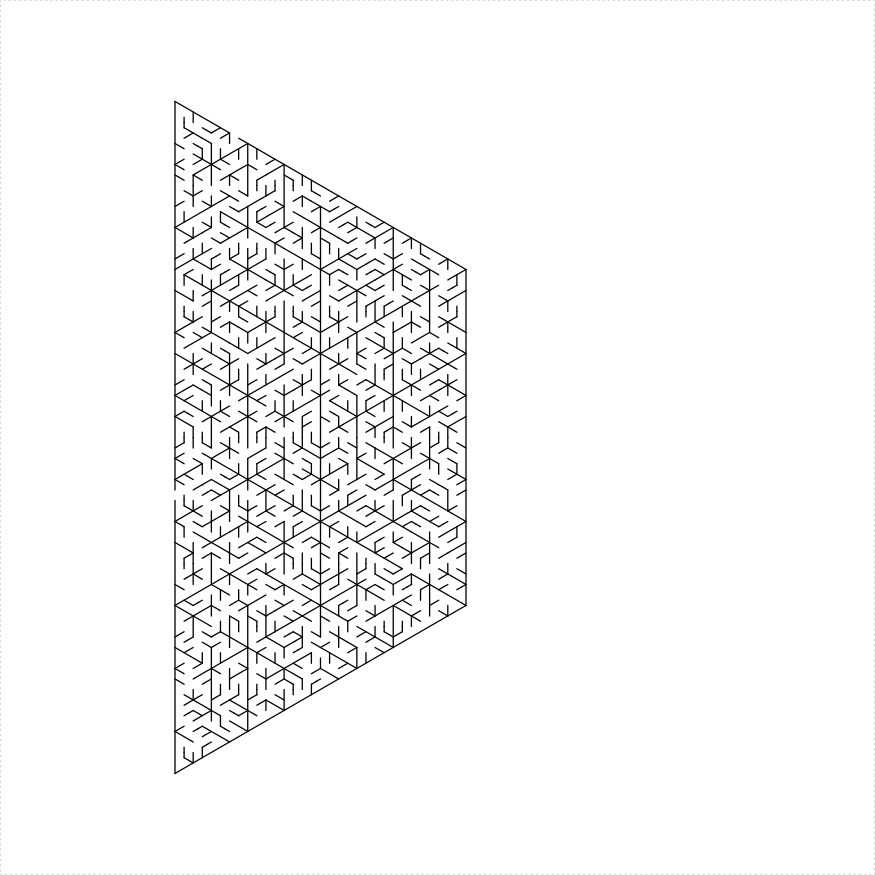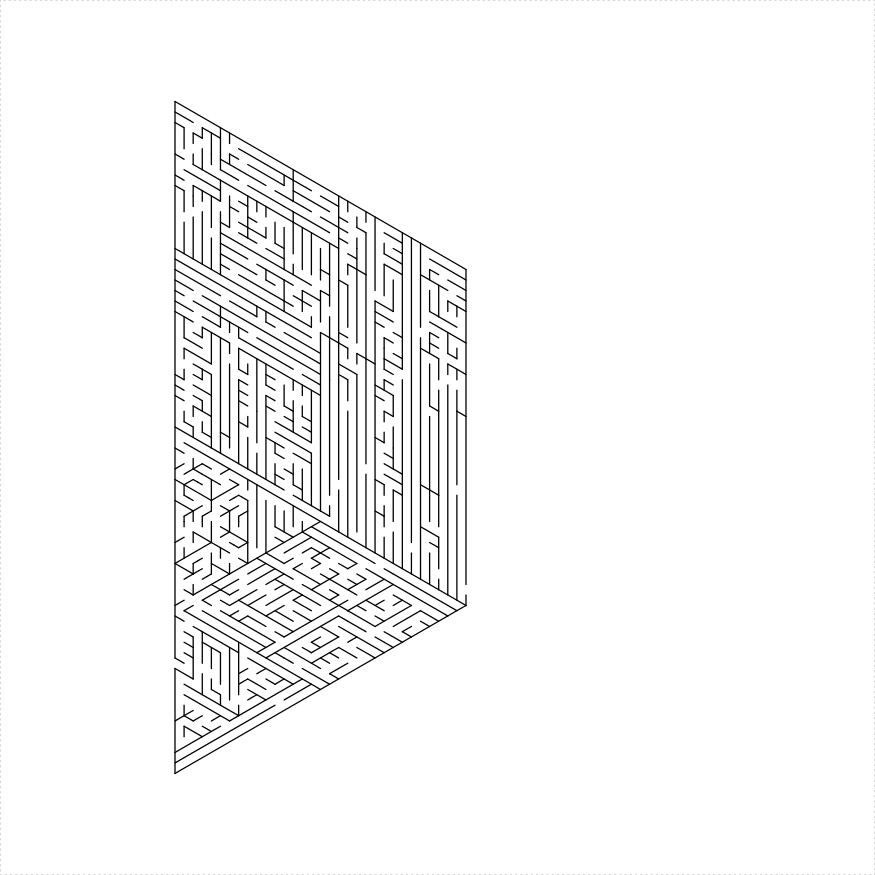# Rhombic Dissections

Regular 2n gons usually admit a dissection into rhombuses. Sometimes, however, these have extremely acute angles, which do not translate into nice mazes. At the moment, there is only support for octagons, and decagons. While a dodecagon would also admit such a dissection, this would require extremely acute angles which would make an ugly maze.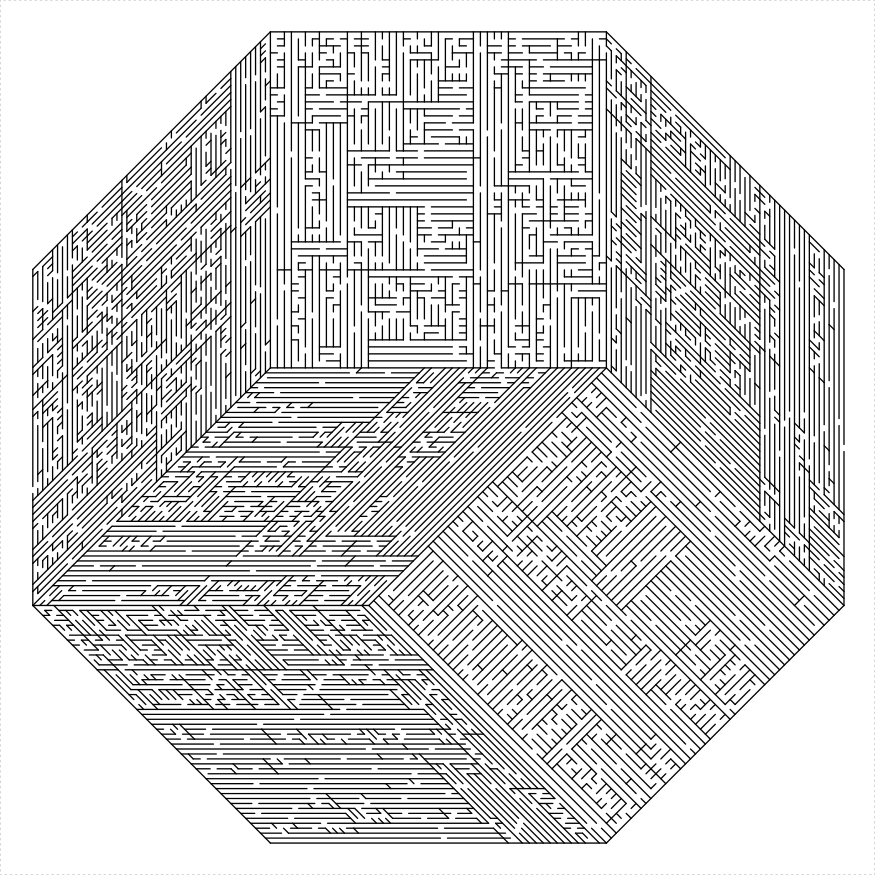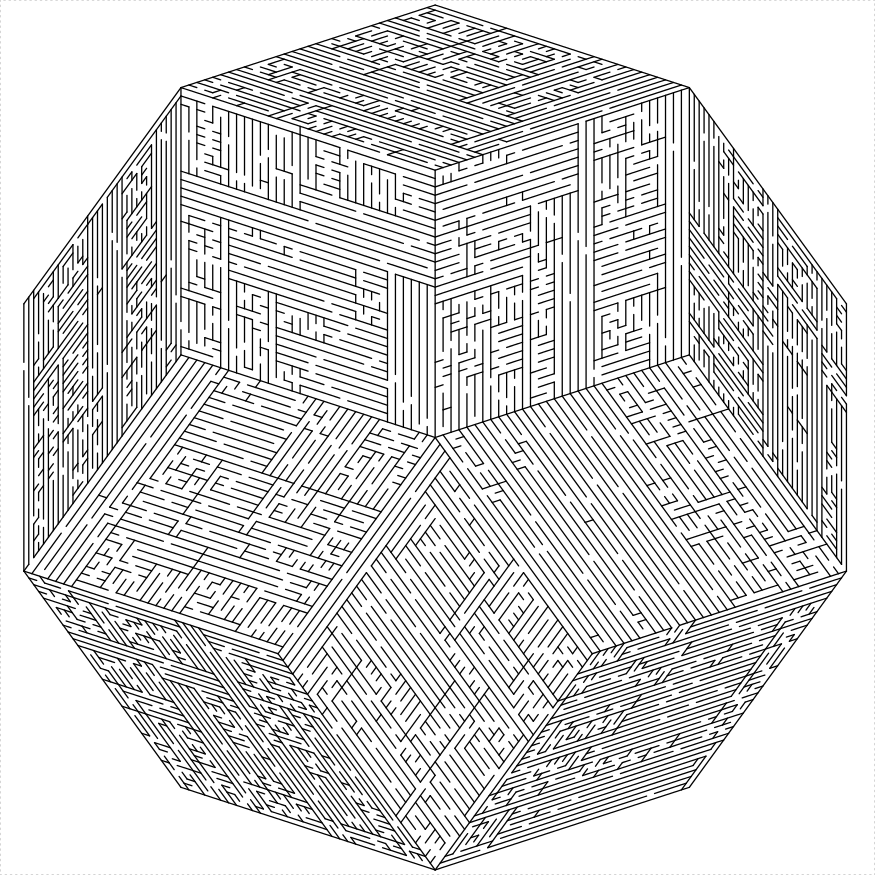# Fractal mazes

## Koch snowflake maze

Everyone's favorite snowflake can also be a maze. Simply fill in triangle bumps with triangular mazes and create lines with holes as needed: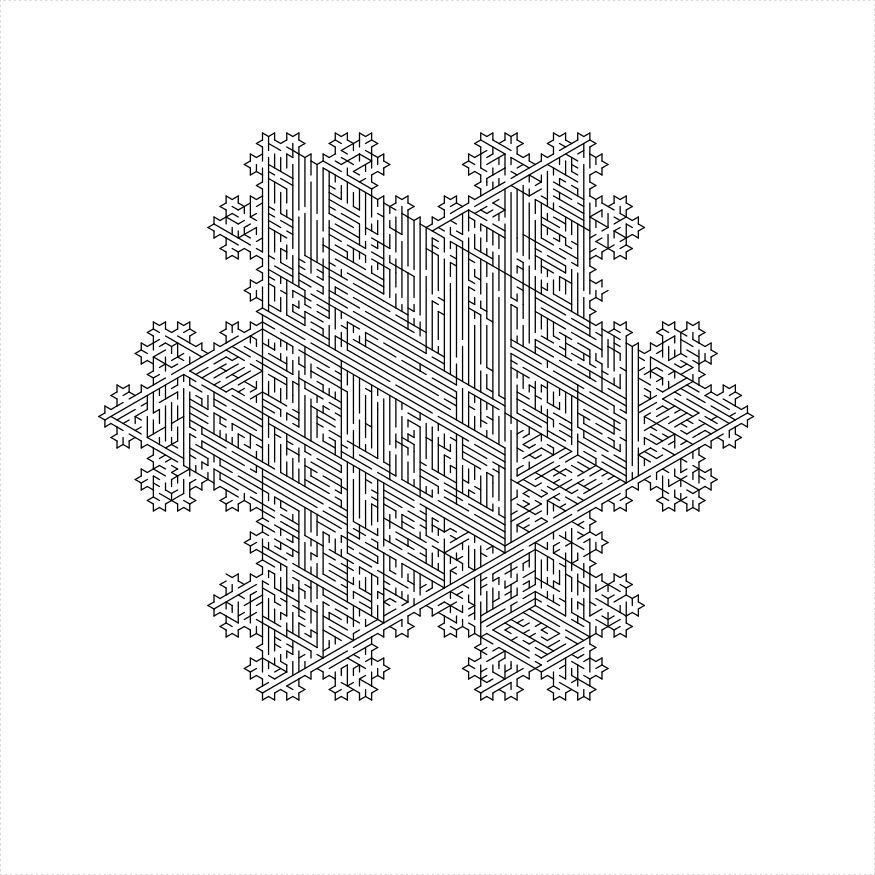Koch flakes of different sizes tile the plane: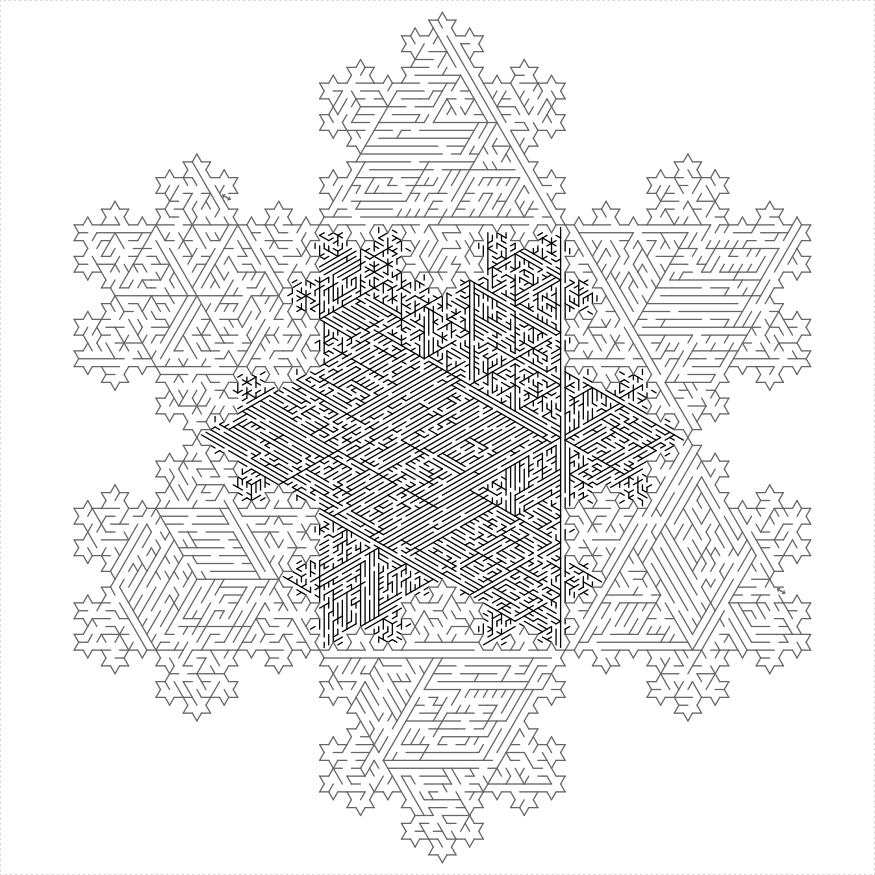## Sierpinski Triangle

Similarly, one can construct a maze in a Sierpinski triangle.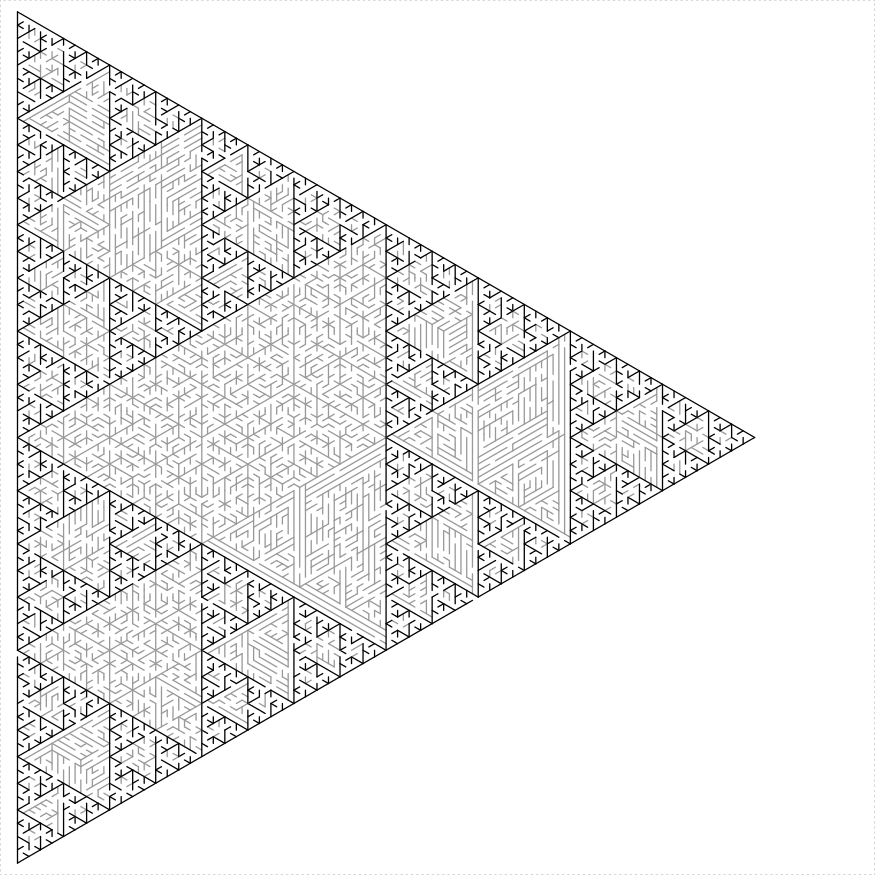And a Sierpinski Carpet: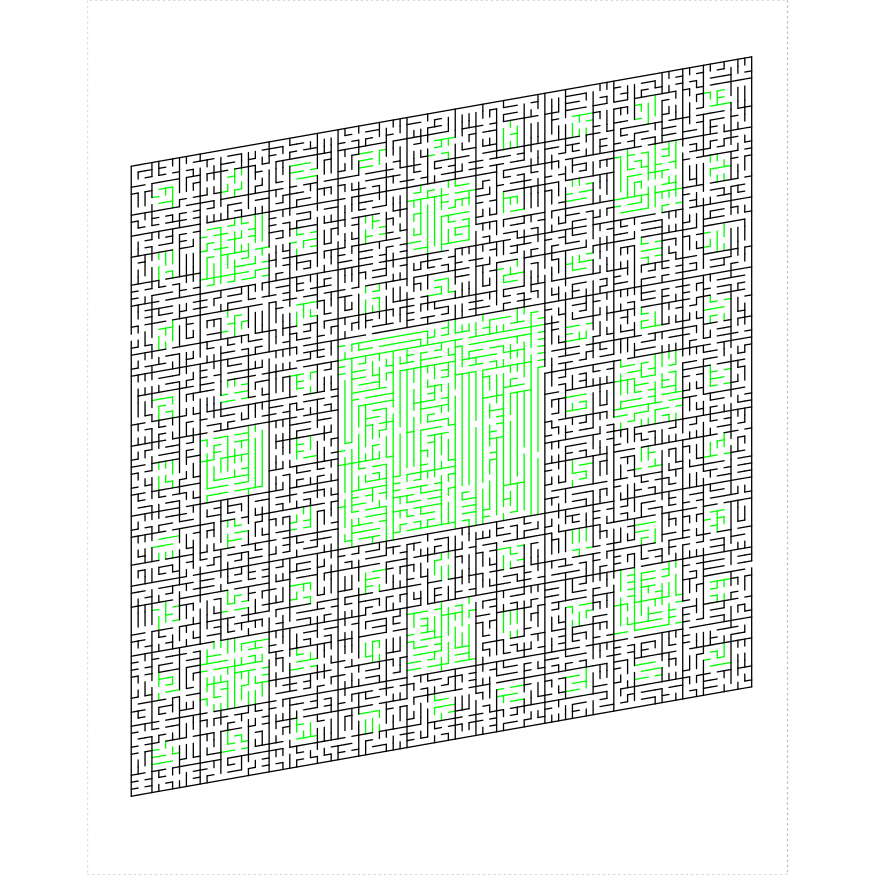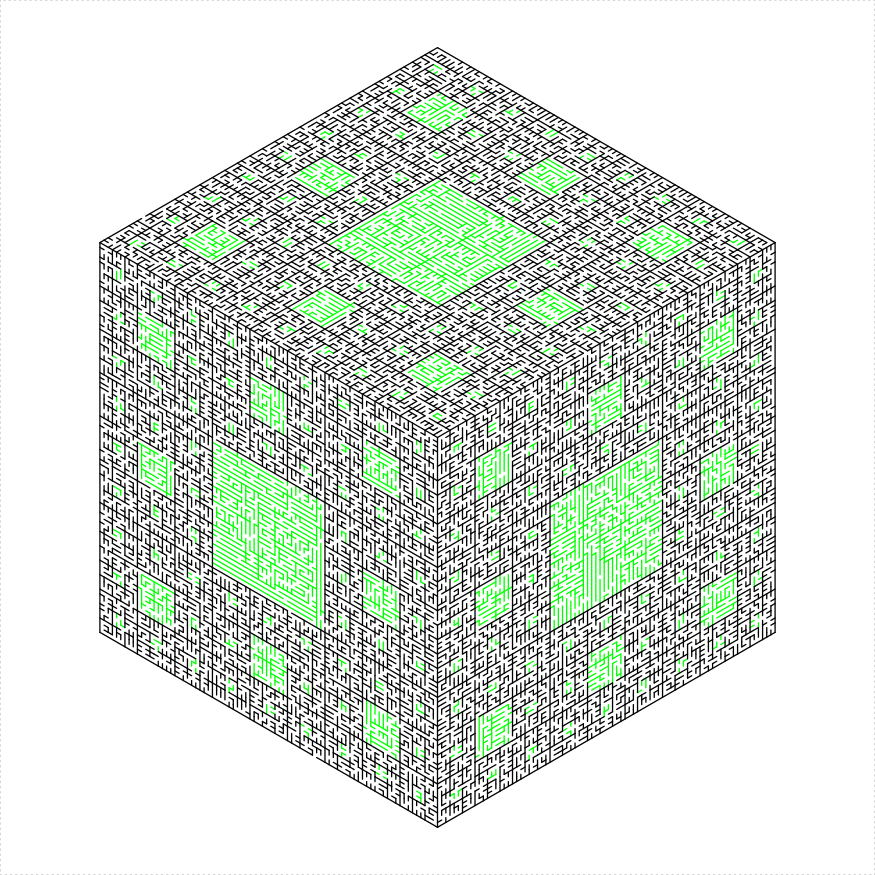One can make four different kinds of Sierpinski trapezoids, the traditional four triangles, a hexaflake, and something like a Dragon fractal: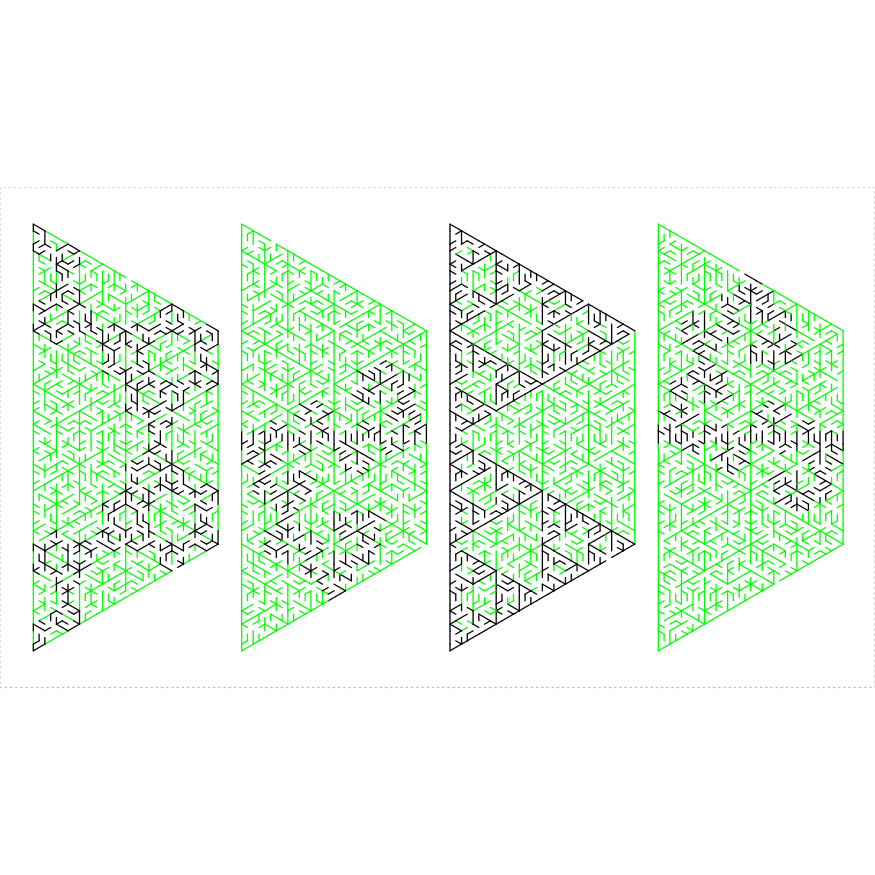## Hexaflake

A hexaflake is a cross between a Koch snowflake and a Sierpinski triangle, at least in theory.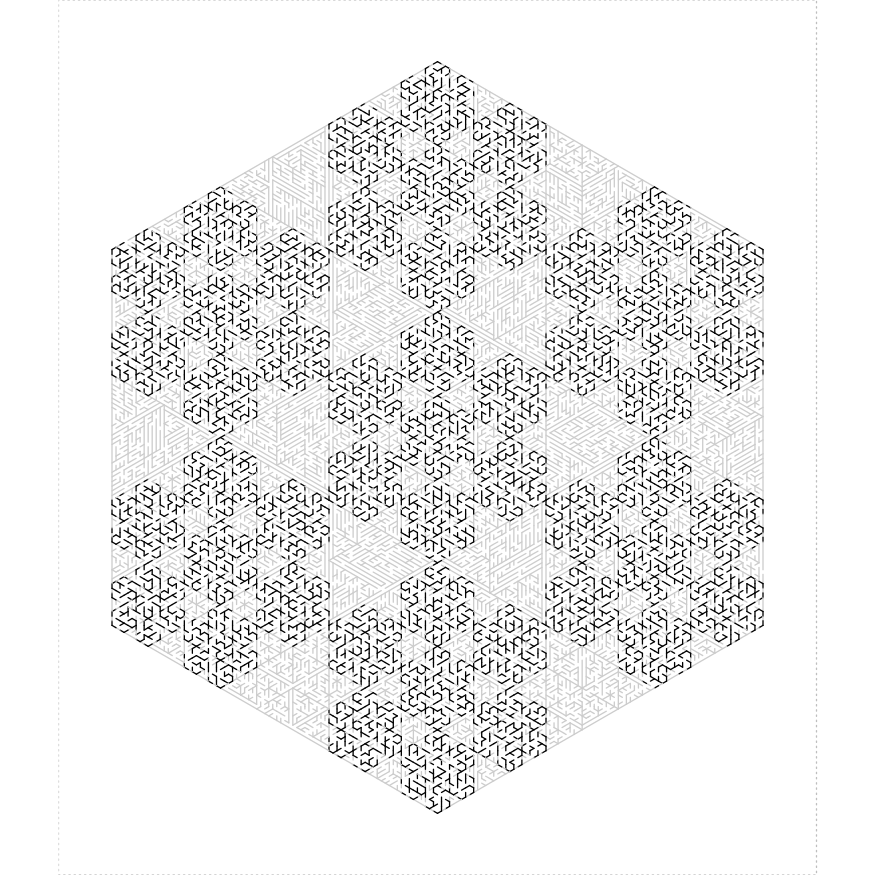# Controls

## unit length

The `unit_len` parameter controls the graphical length of one 'unit', which is the length of holes between sections of the mazes, and is roughly the width of the 'hallways' of a maze. Here is an example of using different unit lengths in a stack of trapezoids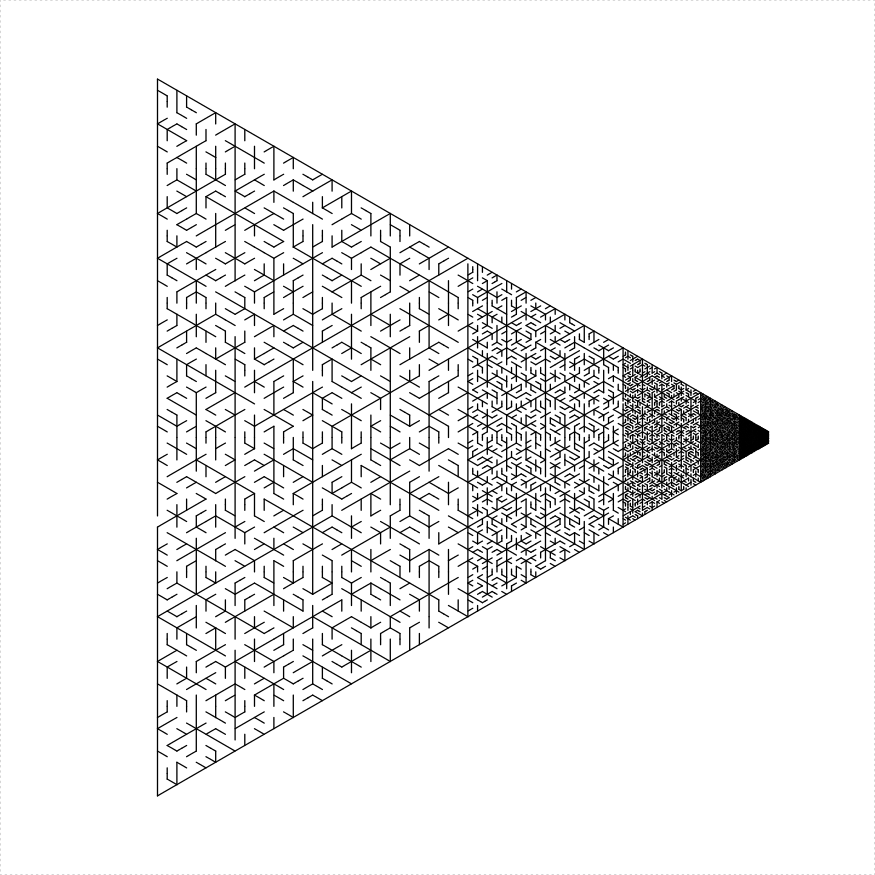## boundaries

The parameters `draw_boundary`, `boundary_lines`, `boundary_holes`, `num_boundary_holes` and `boundary_hole_color` control the drawing of the final outer boundary of polynomial mazes. Without a boundary the maze can be used in recursive construction. Adding a boundary provides the typical entry and exit points of a maze. The parameter `draw_boundary` is a single Boolean that controls whether the boundary is drawn or not. The parameter `boundary_lines` may be a scalar Boolean, or a numeric array giving the indices of which sides should have drawn boundary lines. The sides are numbered in the order in which they appear, and are controlled by the `clockwise` parameter. The parameter `boundary_holes` is a numeric array giving the indices of the boundary lines that should have holes. If `NULL`, then we uniformly choose `num_boundary_holes` holes at random. Holes can be drawn as colored segments with the `boundary_hole_color`, which is a character array giving the color of each hole. The value 'clear' stands in for clear holes.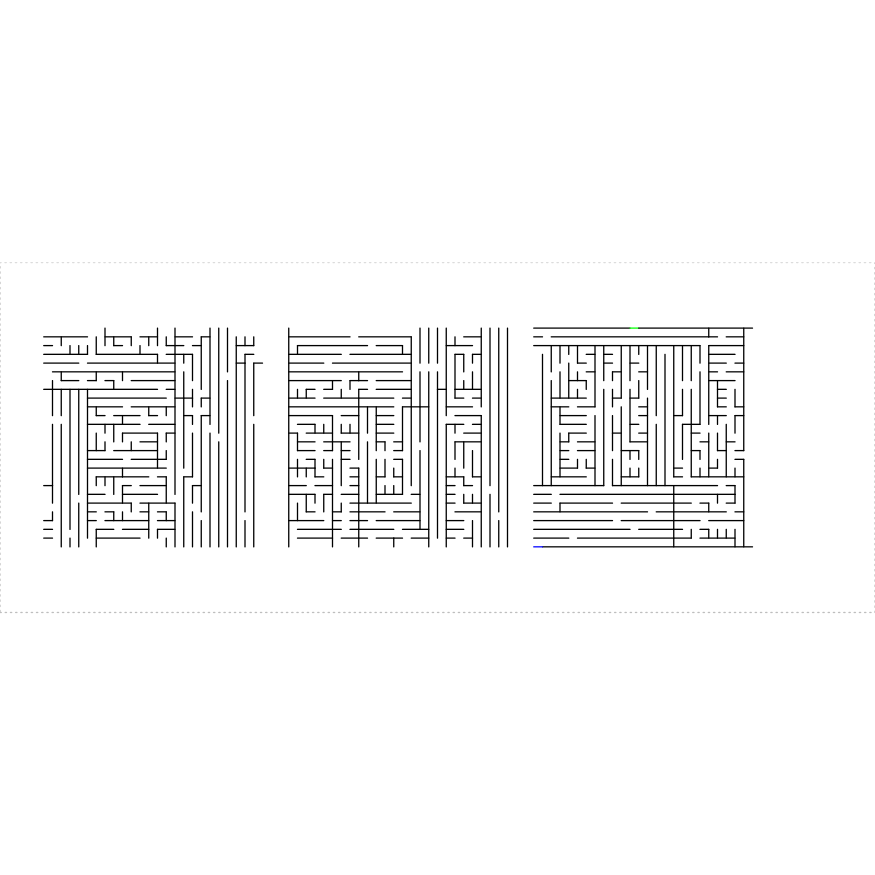## end side

The `end_side` parameter controls which side of the maze the turtle ends on. The default value of 1 essentially causes the turtle to end where it started. The sides are numbered in the order in which the boundary would be drawn. Along with the boundary controls, the ending side can be useful to join together polygons into more complex mazes, as below: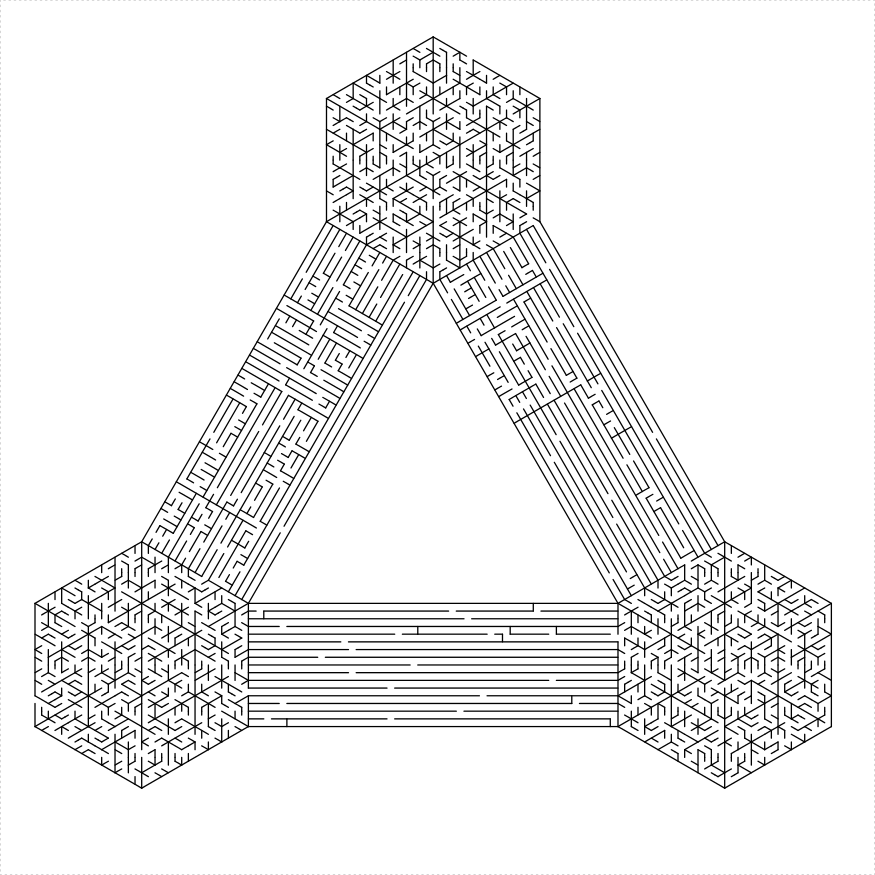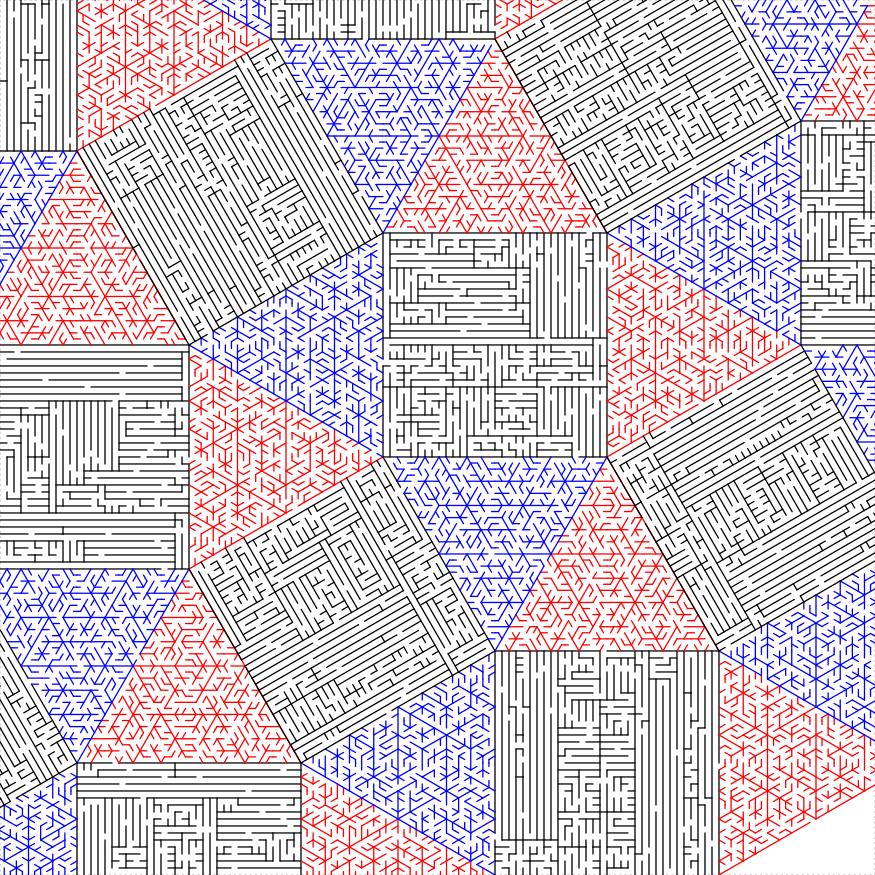# Fun

Or whatever you call it. Here are some mazes built using the primitives.

## A dumb looking tree

Like it says on the label.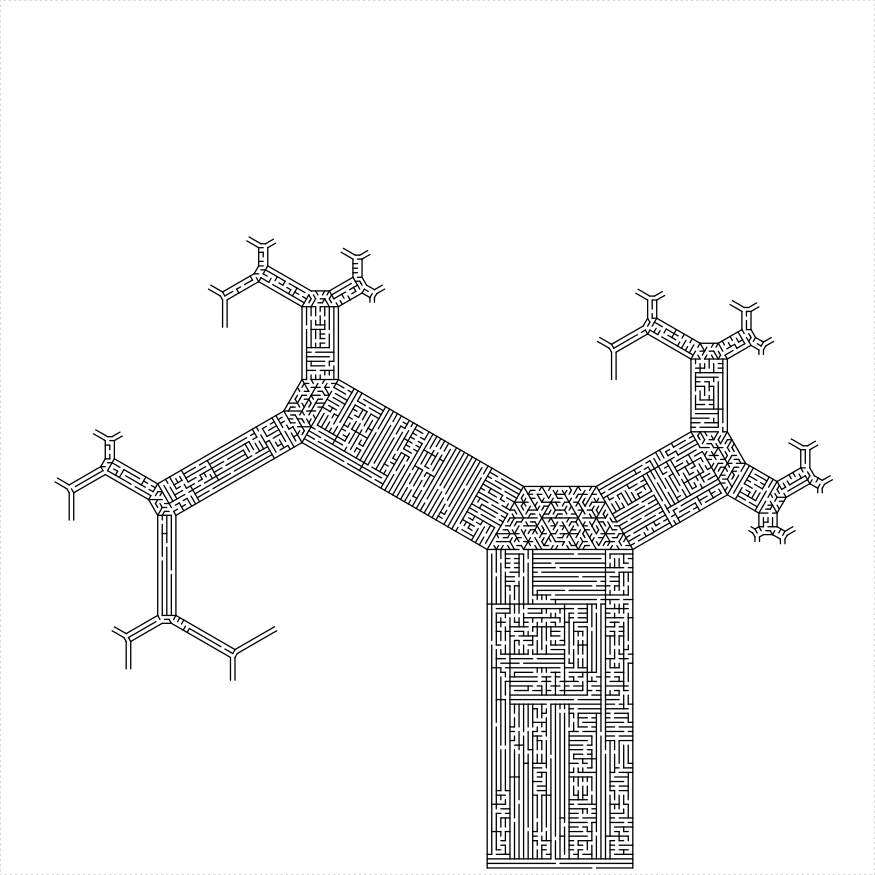## A hex spiral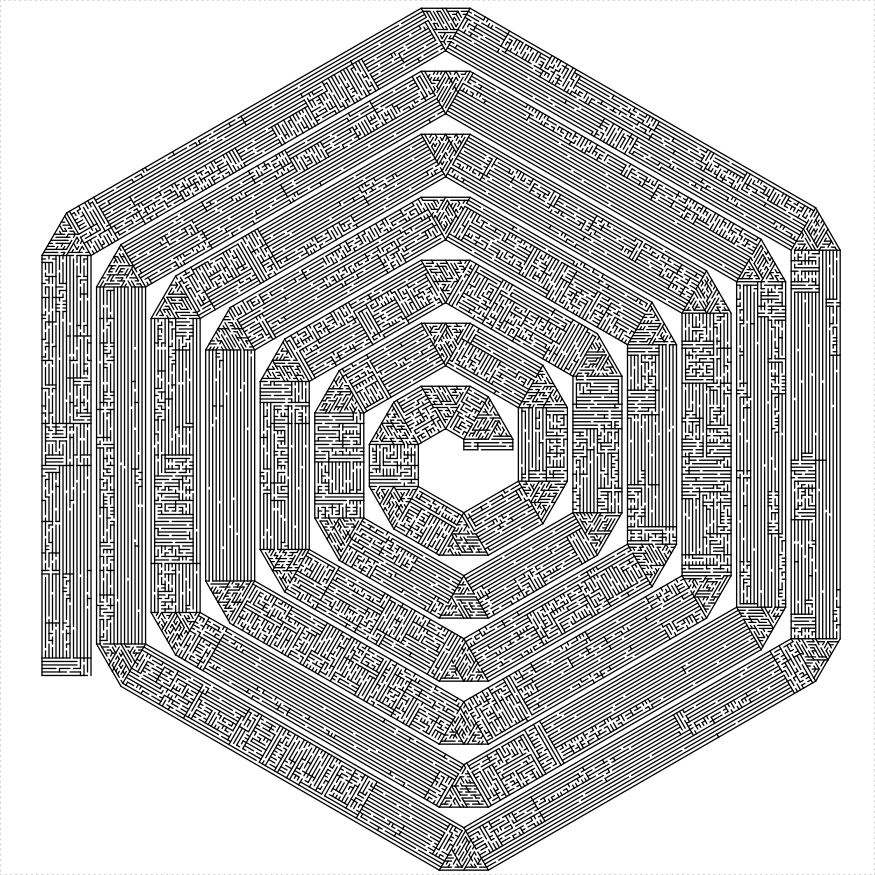## A rectangular spiral

Well, a rhombus spiral.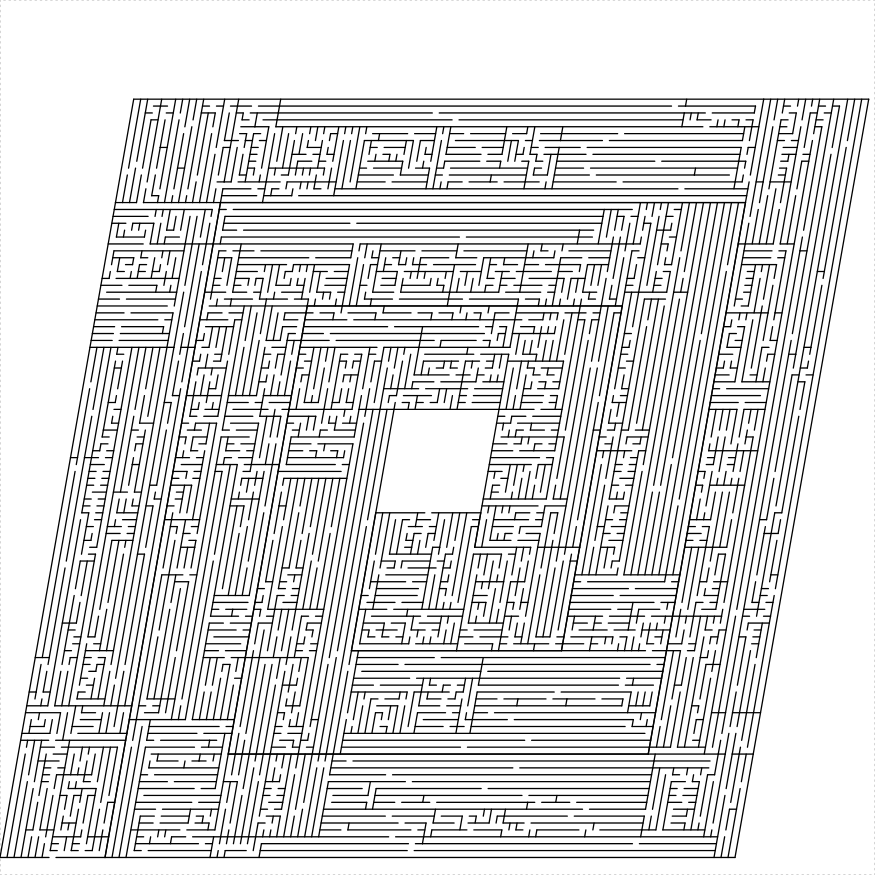## A double rectangular spiral

The path spirals in, then out, joining at the center. This might be buggy.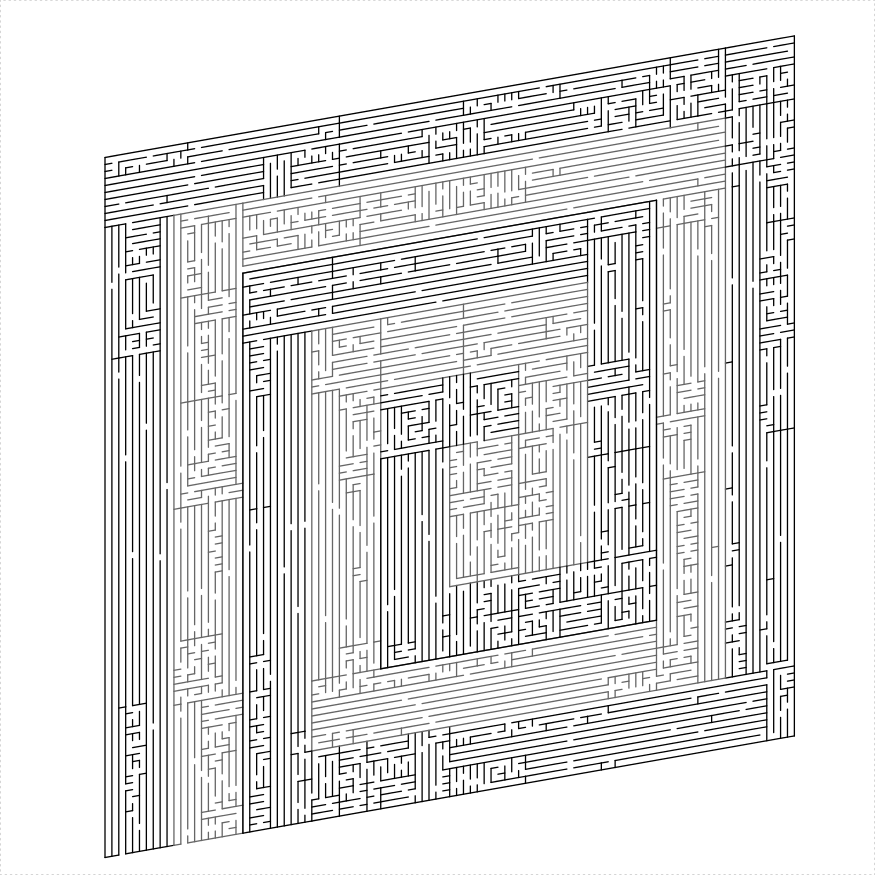## A boustrophedon

As in ox that plods back and forth in a field.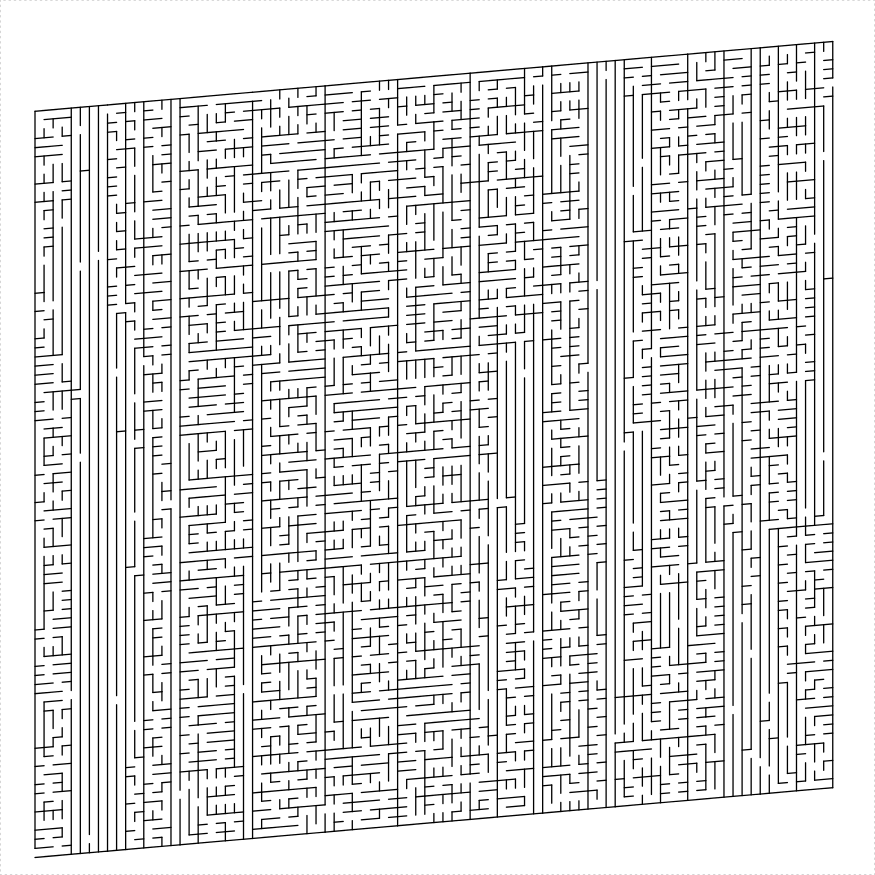# Reference manual

install.packages("mazealls")

0.2.0 by Steven E. Pav, a year ago

https://github.com/shabbychef/mazealls

Report a bug at https://github.com/shabbychef/mazealls/issues

Browse source code at https://github.com/cran/mazealls

Authors: Steven E. Pav [aut, cre]

Documentation:   PDF Manual